## How to mass multiply or divide all values in a column by a number in Excel?

For a list of numbers, you may need to multiply by a certain number for each of them as below screenshot shown. Normally, you need to calculate them one by one with formulas. In this article, we will show you two methods to quickly multiply or divide all values in a column by a number at once in details.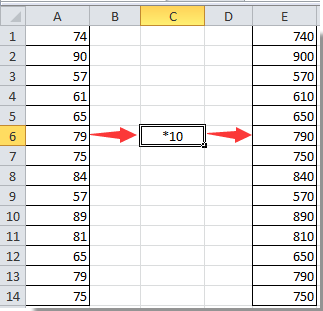#### Mass multiply or divide all values in a column by a number in Excel

You can multiply or divide all values in a column by a certain number as follows.

1. Enter the certain number in a blank cell (for example, you need to multiply or divide all values by number 10, then enter number 10 into the blank cell). Copy this cell with pressing the Ctrl + C keys simultaneously.

2. Select the number list you need to batch multiply, then click Home > Paste > Paste Special. See screenshot: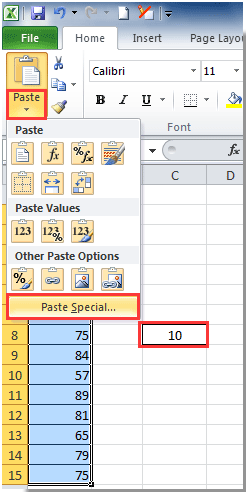3. In the Paste Special dialog box, select the Multiply or Divide option in the Operation section as you need (here we select the Multiply option), and then click the OK button.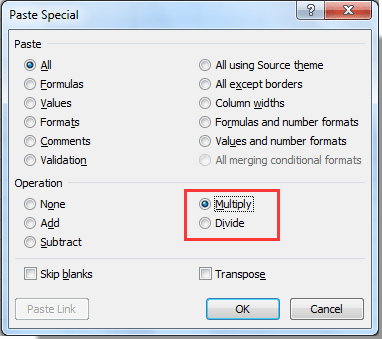You can see the operation result as below screenshot shown.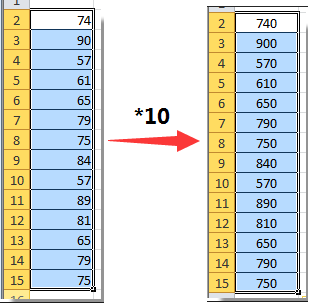#### Easily multiply or divide all values in a column by a number with Kutools for Excel

The Operation tool of Kutools for Excel will help you easily mass multiply or divide all values in a column or range by a certain number in Excel. Please browse for more details.

1. Select the column with values you need to batch multiply or divide by a number, then click Kutools > More > Operation. See screenshot: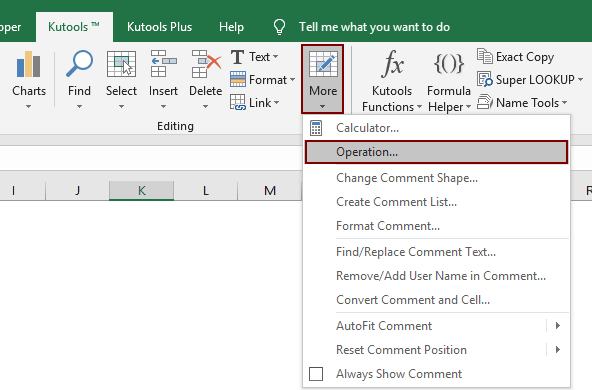2. In the Operation Tools dialog box, select the operation type you need in the Operation box (here we select Multiplication option), enter the certain number for the multiplication operand into the textbox, and then click the OK button.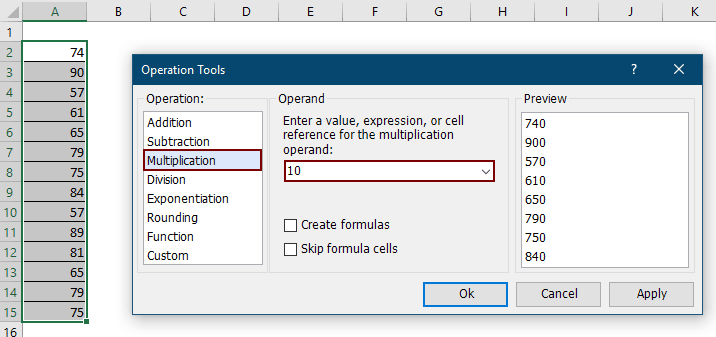Then all selected cells are multiplied with a certain number at once.

Notes:

• You can create formulas for the list with checking the Create formulas box.
• If there already have formulas in the list, you can skip calculating these cells by checking the Skip formula cells box.

If you want to have a free trial (30-day) of this utility, please click to download it, and then go to apply the operation according above steps.

### Best Office Productivity Tools

 Popular Features: Find, Highlight or Identify Duplicates   |  Delete Blank Rows   |  Combine Columns or Cells without Losing Data   |   Round without Formula ... Super Lookup: Multiple Criteria VLookup  |   Multiple Value VLookup  |   VLookup Across Multiple Sheets   |   Fuzzy Lookup .... Advanced Drop-down List: Quickly Create Drop Down List   |  Dependent Drop Down List   |  Multi-select Drop Down List .... Column Manager: Add a Specific Number of Columns  |  Move Columns  |  Toggle Visibility Status of Hidden Columns  |  Compare Ranges & Columns ... Featured Features: Grid Focus   |  Design View   |   Big Formula Bar   |  Workbook & Sheet Manager   |  Resource Library (Auto Text)   |  Date Picker   |  Combine Worksheets   |  Encrypt/Decrypt Cells   |  Send Emails by List   |  Super Filter   |   Special Filter (filter bold/italic/strikethrough...) ... Top 15 Toolsets:  12 Text Tools (Add Text, Remove Characters, ...)   |   50+ Chart Types (Gantt Chart, ...)   |   40+ Practical Formulas (Calculate age based on birthday, ...)   |   19 Insertion Tools (Insert QR Code, Insert Picture from Path, ...)   |   12 Conversion Tools (Numbers to Words, Currency Conversion, ...)   |   7 Merge & Split Tools (Advanced Combine Rows, Split Cells, ...)   |   ... and more

Supercharge Your Excel Skills with Kutools for Excel, and Experience Efficiency Like Never Before. Kutools for Excel Offers Over 300 Advanced Features to Boost Productivity and Save Time.  Click Here to Get The Feature You Need The Most...#### Office Tab Brings Tabbed interface to Office, and Make Your Work Much Easier

• Enable tabbed editing and reading in Word, Excel, PowerPoint, Publisher, Access, Visio and Project.
• Open and create multiple documents in new tabs of the same window, rather than in new windows.
• Increases your productivity by 50%, and reduces hundreds of mouse clicks for you every day!Rated 0.5 out of 5 · 1 ratings
This comment was minimized by the moderator on the site
I need to divide a set amount of money between a row of numbers so that the total is the set number. IE, I have \$5,000 to divide between 15 people so that each gets the same amount of money that totals up to \$5,000. How do I do that?
This comment was minimized by the moderator on the site
Okay, so 4 pages of useless instructions? How do I divide the sum of 3 rows with a SINGLE NUMBER, such as B22:B24 "divided by 2" rather than some other stupid row? Why is this stupid application so bloody complicated?!
Rated 0.5 out of 5
This comment was minimized by the moderator on the site
Hi,
I don't particularly understand what you mean. If you want to divide the sum of every 3 rows in a column by a single number, such as the example you provided. The following formula can do you a favor. After getting the first result, use the AutoFill Handle to get the rest of the results.
=SUM(OFFSET(\$B\$22,(ROW()-ROW(\$B\$22))*3,0,3,1))/2
This comment was minimized by the moderator on the site
Hello,

I am trying to create a formula that will multiply certain numbers within a range (1-9) by specific numbers. For example if there is a 1 in a cell, I would like that to be multiplied by 24, if there is the number 2 in a cell I would like that to be multiplied by 13.5, if the number 3 is found in a cell I would like that to be multiplied by 10, the number 4 to be multiplied by 8.5, number 5 multiplied by 7.4, number 6 multiplied by 6.67, number 7 multiplied by 6.29, number 8 multiplied by 5.88, and number 9 multiplied by 5.56.

Essentially I am looking for this formula to convert numbers 1-9 to a scale of 50 and I am struggling with how to do that using conventional formulas found in excel or google sheets.

Anything you can do to help would be greatly appreciated. Thank you!
There are no comments posted here yet